Miscellaneous

Chapter 4 Class 12 Determinants
Serial order wise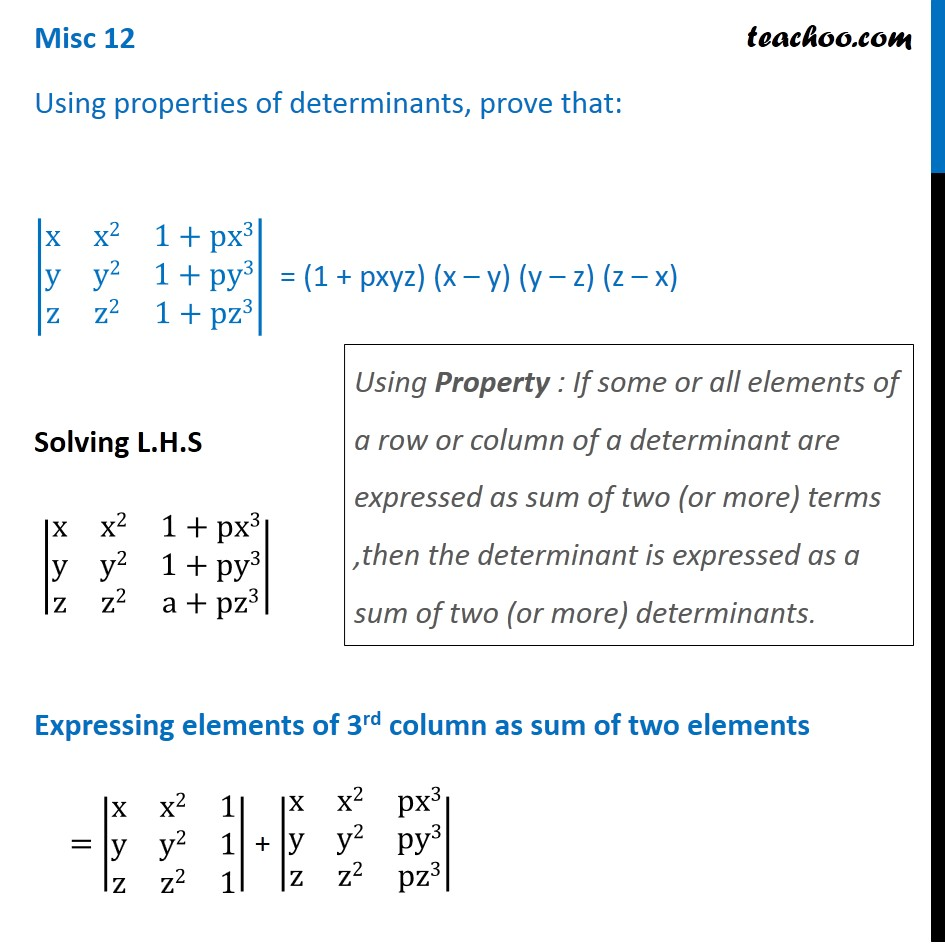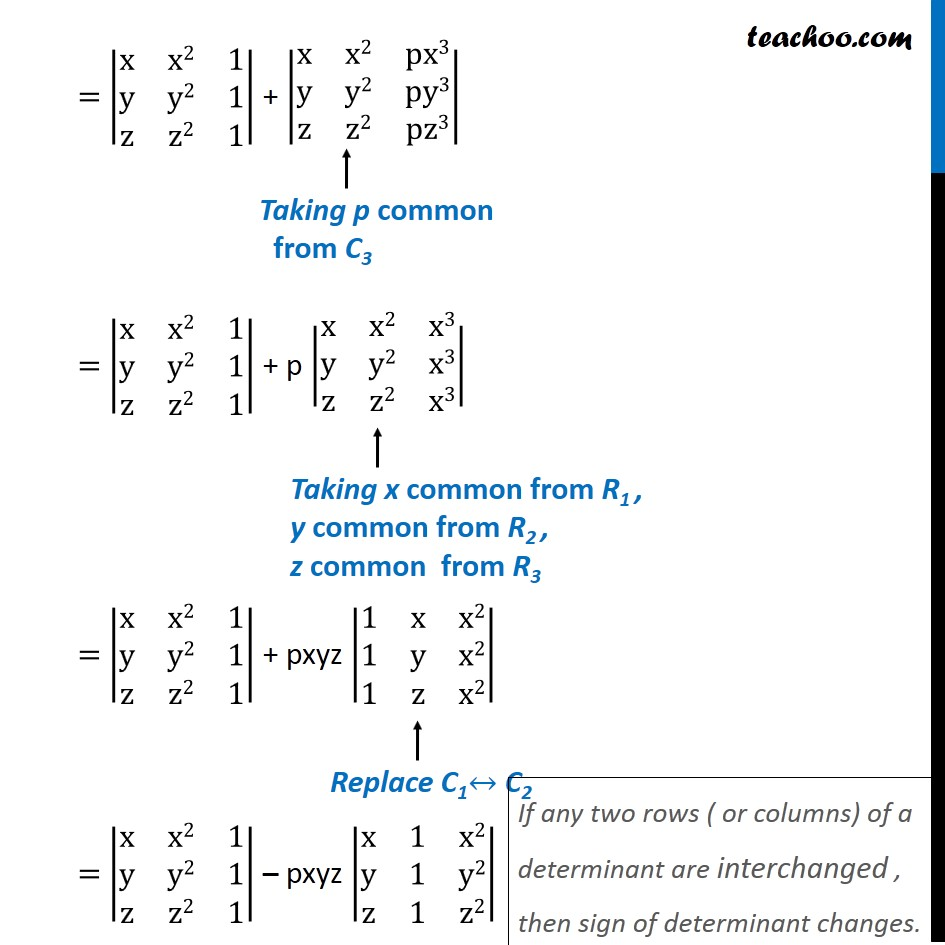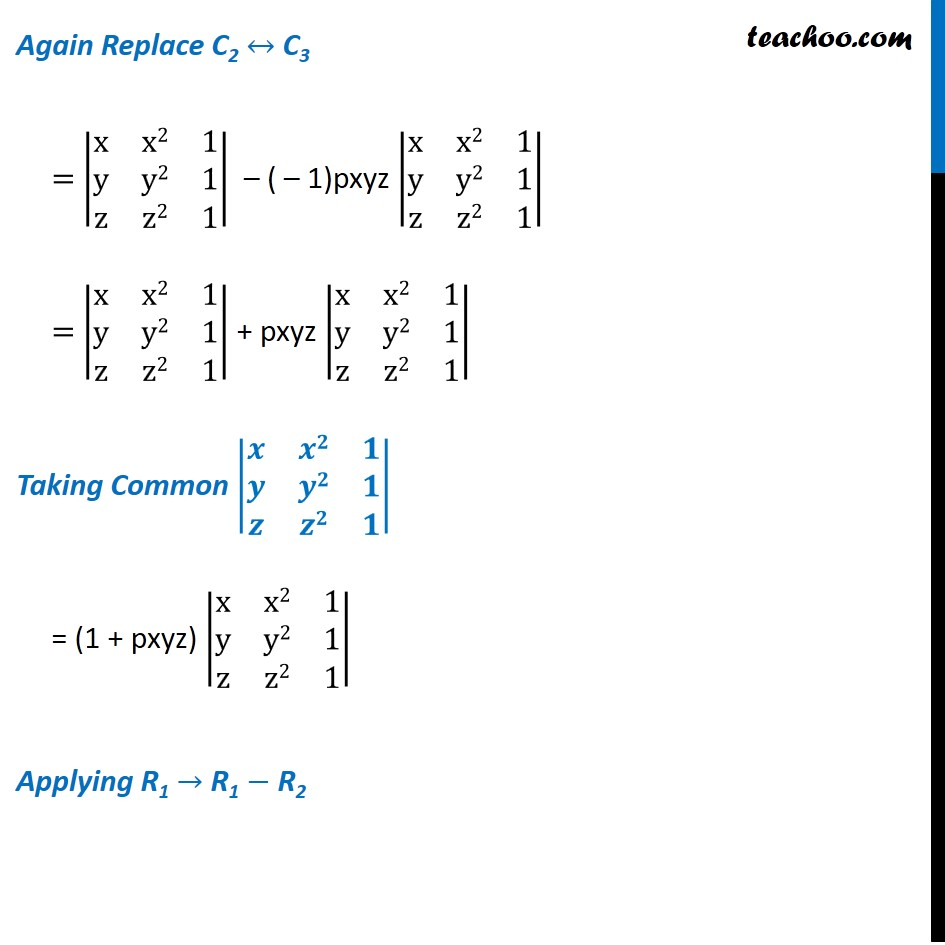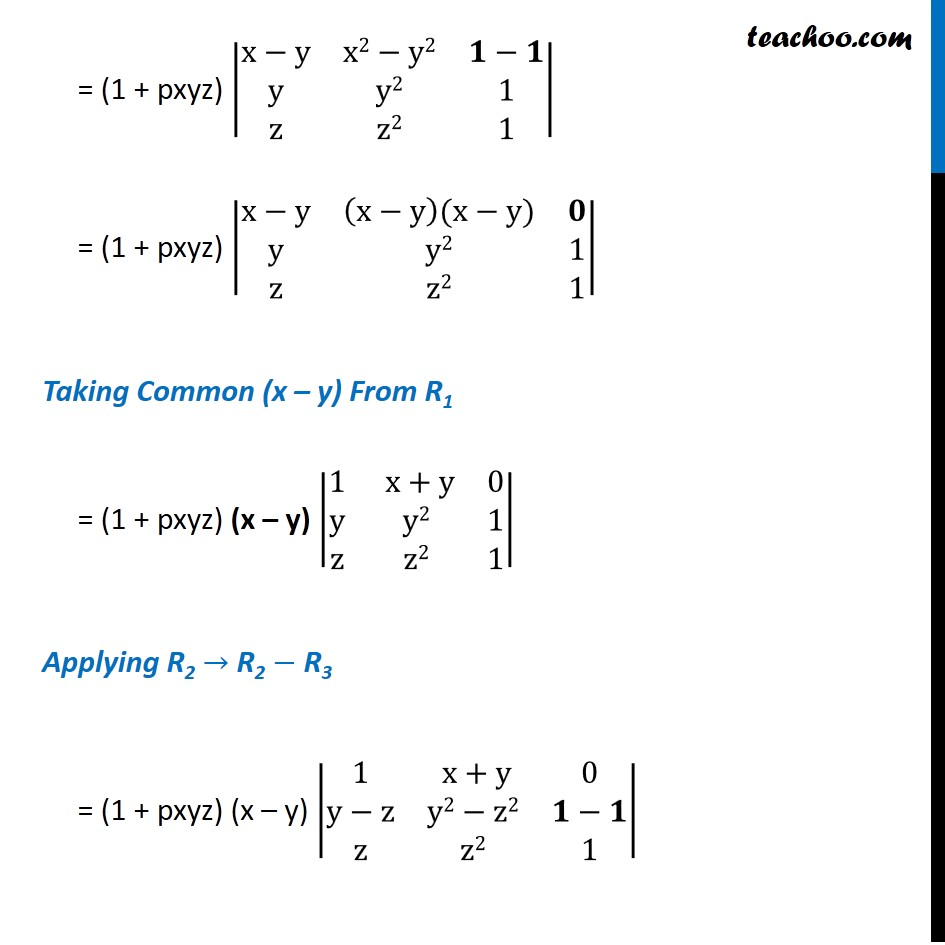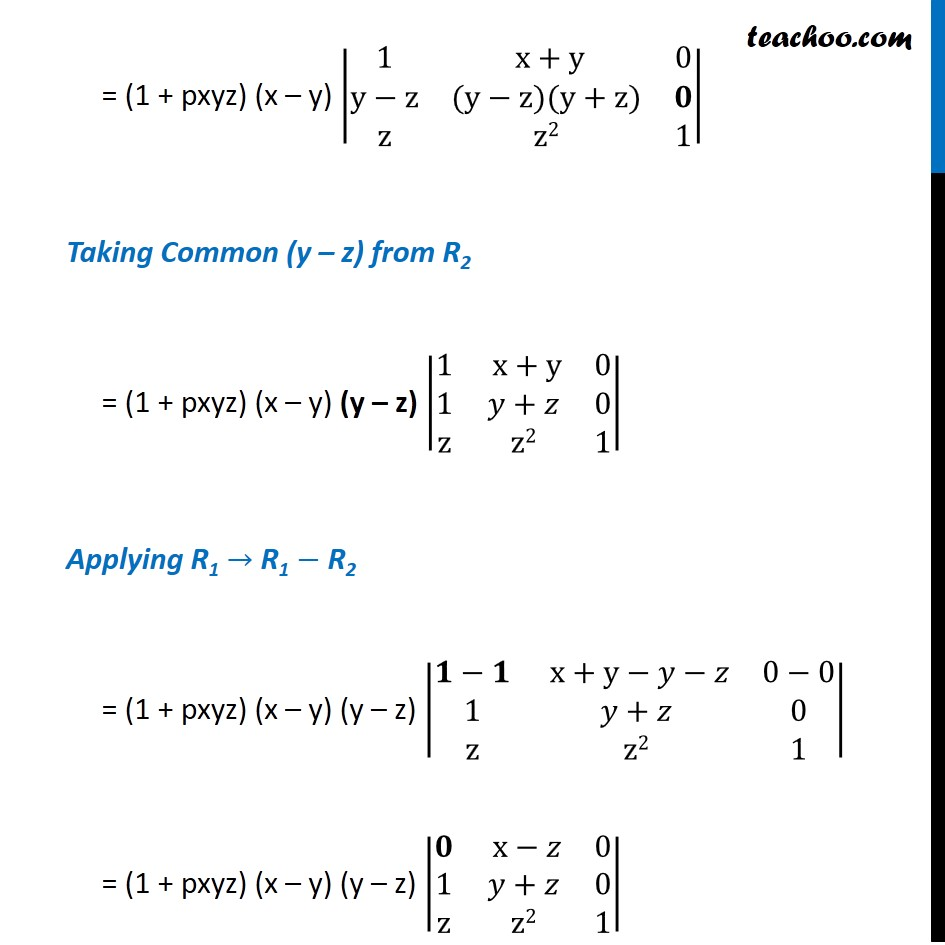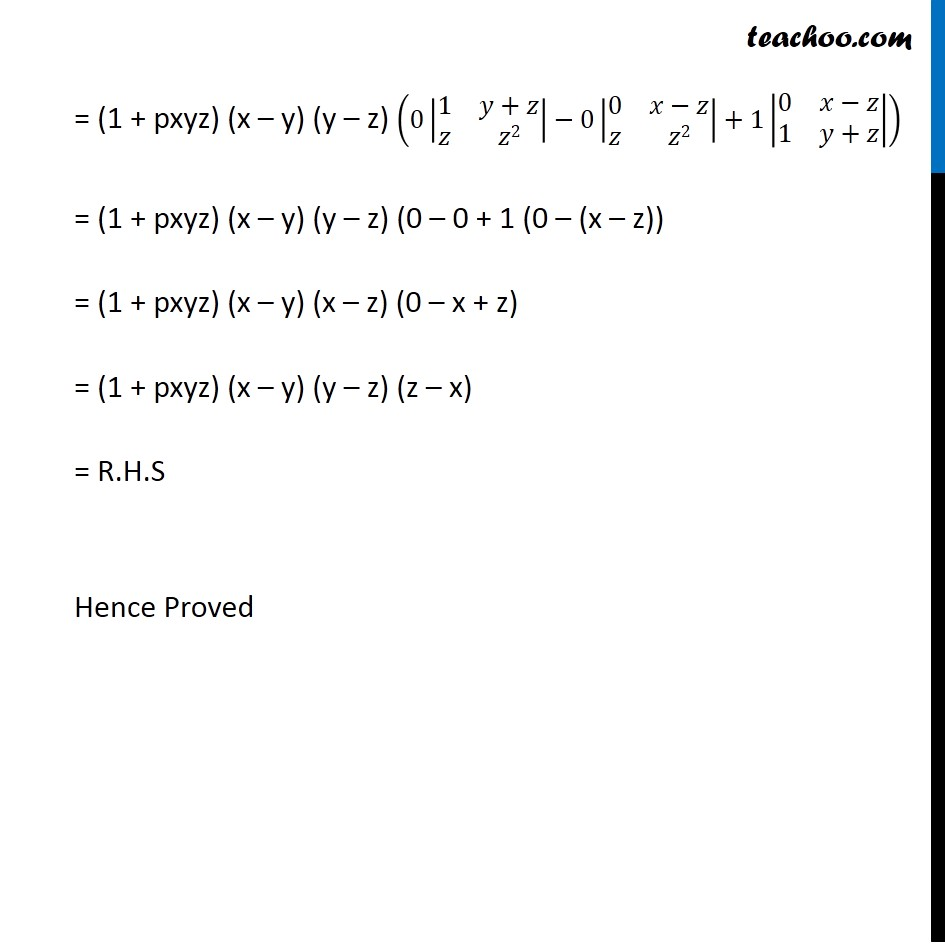Learn in your speed, with individual attention - Teachoo Maths 1-on-1 Class

### Transcript

Question 6 Using properties of determinants, prove that: |■8(x&x2&1+px3@y&y2&1+py3@z&z2&1+pz3)| = (1 + pxyz) (x – y) (y – z) (z – x) Solving L.H.S |■8(x&x2&1+px3@y&y2&1+py3@z&z2&a+pz3)| Expressing elements of 3rd column as sum of two elements = |■8(x&x2&1@y&y2&1@z&z2&1)| + |■8(x&x2&px3@y&y2&py3@z&z2&pz3)| Using Property : If some or all elements of a row or column of a determinant are expressed as sum of two (or more) terms ,then the determinant is expressed as a sum of two (or more) determinants. = |■8(x&x2&1@y&y2&1@z&z2&1)| + |■8(x&x2&px3@y&y2&py3@z&z2&pz3)| = |■8(x&x2&1@y&y2&1@z&z2&1)| + p |■8(x&x2&x3@y&y2&x3@z&z2&x3)| = |■8(x&x2&1@y&y2&1@z&z2&1)| + pxyz |■8(1&x&x2@1&y&x2@1&z&x2)| = |■8(x&x2&1@y&y2&1@z&z2&1)| – pxyz |■8(x&1&x2@y&1&y2@z&1&z2)| Taking p common from C3 Taking x common from R1 , y common from R2 , z common from R3 If any two rows ( or columns) of a determinant are interchanged , then sign of determinant changes. Again Replace C2 ↔ C3 = |■8(x&x2&1@y&y2&1@z&z2&1)| – ( – 1)pxyz |■8(x&x2&1@y&y2&1@z&z2&1)| = |■8(x&x2&1@y&y2&1@z&z2&1)| + pxyz |■8(x&x2&1@y&y2&1@z&z2&1)| Taking Common |■8(𝒙&𝒙𝟐&𝟏@𝒚&𝒚𝟐&𝟏@𝒛&𝒛𝟐&𝟏)| = (1 + pxyz) |■8(x&x2&1@y&y2&1@z&z2&1)| Applying R1 → R1 − R2 = (1 + pxyz) |■8(x−y&x2−y2&𝟏−𝟏@y&y2&1@z&z2&1)| = (1 + pxyz) |■8(x−y&(x−y)(x−y)&𝟎@y&y2&1@z&z2&1)| Taking Common (x – y) From R1 = (1 + pxyz) (x – y) |■8(1& x+y&0@y&y2&1@z&z2&1)| Applying R2 → R2 − R3 = (1 + pxyz) (x – y) |■8(1& x+y&0@y−z&y2−z2&𝟏−𝟏@z&z2&1)| = (1 + pxyz) (x – y) |■8(1& x+y&0@y−z&(y−z)(y+z)&𝟎@z&z2&1)| Taking Common (y – z) from R2 = (1 + pxyz) (x – y) (y – z) |■8(1& x+y&0@1&𝑦+𝑧&0@z&z2&1)| Applying R1 → R1 − R2 = (1 + pxyz) (x – y) (y – z) |■8(𝟏−𝟏& x+y−𝑦−𝑧&0−0@1&𝑦+𝑧&0@z&z2&1)| = (1 + pxyz) (x – y) (y – z) |■8(𝟎& x−𝑧&0@1&𝑦+𝑧&0@z&z2&1)| Expanding determinant along C3 = (1 + pxyz) (x – y) (y – z) (0|■8(1&𝑦+𝑧@𝑧&𝑧2)|−0|■8(0&𝑥−𝑧@𝑧&𝑧2)|+1|■8(0&𝑥−𝑧@1&𝑦+𝑧)|) = (1 + pxyz) (x – y) (y – z) (0 – 0 + 1 (0 – (x – z)) = (1 + pxyz) (x – y) (x – z) (0 – x + z) = (1 + pxyz) (x – y) (y – z) (z – x) = R.H.S Hence Proved